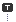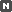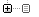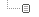Every unexpected event is a path to learning for you.

### 카테고리분류 전체보기 (2328)Unity3D (572)Programming (472)Unreal (4)Gamebryo (56)Tip & Tech (185)협업 (34)3DS Max (3)Game (12)Utility (116)Etc (92)Portfolio (19)Subject (90)iOS,OSX (38)Android (13)Linux (5)잉여 프로젝트 (2)게임이야기 (1)Memories (19)Interest (37)Thinking (36)한글 (26)PaperCraft (5)Animation (408)Wallpaper (2)재테크 (19)Exercise (3)나만의 맛집 (2)냥이 (9)육아 (9)
Total1,346,280
Today86
Yesterday132### 달력

« » 2019.12
1 2 3 4 5 6 7
8 9 10 11 12 13 14
15 16 17 18 19 20 21
22 23 24 25 26 27 28
29 30 31

### 'nullable'에 해당되는 글 2건

1. 2014.08.18 Nullable Types
2. 2012.01.19 Nullable 형식 사용

## Nullable Types

Unity3D/Extensions / 2014. 8. 18. 02:29

## Nullable Types

##### Posted by David Laskey on January 14th, 2014

Sometimes you have variables that have important information but only after certain game events occur. For example: A character in your game may be idle until they’re told to go to an assigned destination.

 `public` `class` `Character : MonoBehaviour ``{``    ``Vector3 targetPosition;` `    ``void` `MoveTowardsTargetPosition()``    ``{``        ``if``(targetPosition != Vector3.zero)``        ``{``            ``//Move towards the target position!``        ``}``    ``}` `    ``public` `void` `SetTargetPosition(Vector3 newPosition)``    ``{``        ``targetPosition = newPosition;``    ``}``}`

In this case, we want the character to move towards the target position only if it’s been assigned. In the code above, we do this by just checking if targetPosition is not equal to its default value (0, 0, 0).  But now we have an issue: what if you want your character to move to (0, 0, 0)? You don’t want to discredit the possibility of that value being used because it might come up sometime during the game!

Luckily, there’s a trick to help avoid comparing arbitrary values for confirming that a variable has been initialized: Nullable Types.

## Using Nullable Types

To make a nullable type, just add a “?” after the type declaration of any variable that is a Value Type (eg. Vector3, Rect, int, float).

 `public` `class` `Character : MonoBehaviour ``{``    ``//Notice the added "?"``    ``Vector3? targetPosition;` `    ``void` `MoveTowardsTargetPosition()``    ``{``        ``if` `(targetPosition.HasValue)``        ``{``            ``//Move towards the target position!``            ``//use targetPosition.Value for the actual value``        ``}``    ``}` `    ``public` `void` `SetTargetPosition(Vector3 newPosition)``    ``{``        ``targetPosition = newPosition;``    ``}``}`

Seen here, nullable types have two properties we can use: HasValue (true if the variable has been assigned, false otherwise), and Value (the actual assigned value of the variable).

 `//First, check if the variable has been assigned a value``if` `(targetPosition.HasValue)``{``    ``//move towards targetPosition.Value``}``else``{``    ``//targetPosition.Value is invalid! Don't use it!``}`

## Usage Notes

• To revert a nullable type to having “no value”, set it to null
• You can NOT create nullable types from classes, or reference types (they can already be set to null)

As usual, if you have any questions or tips to add, do so in the comments below!

#### 'Unity3D > Extensions' 카테고리의 다른 글

 일괄적으로 Texture Import Setting 변경  (0) 2015.01.30 2014.08.18 2014.08.18 2014.04.22 2014.04.02 2014.01.12

## Nullable 형식 사용

Programming/C# / 2012. 1. 19. 11:33
Visual Studio 20051명 중 1명이 도움이 되는 것으로 평가 이 항목 평가

nullable 형식을 사용하면 내부 형식의 값을 모두 나타낼 수 있을 뿐만 아니라 null 값을 추가로 나타낼 수 있습니다. nullable 형식은 다음과 같은 두 가지 방법 중 하나로 선언됩니다.

System.Nullable<T> variable

- 또는 -

T? variable

T는 nullable 형식의 내부 형식입니다. T는 struct를 비롯한 임의의 값 형식이 될 수 있지만 참조 형식은 될 수 없습니다.

nullable 형식이 필요한 경우를 예로 들면, true와 false라는 두 가지 값만 갖는 일반적인 부울 변수를 생각해 볼 수 있습니다. 이런 변수에는 "정의되지 않은 상태"를 의미하는 값이 없습니다. 여러 프로그래밍 응용 프로그램에서 변수는 정의되지 않은 상태로 있을 수 있으며, 데이터베이스 상호 작용이 가장 대표적인 예입니다. 예를 들어, 데이터베이스의 필드는 true나 false 값을 포함할 수 있지만 값을 전혀 포함하지 않을 수 있습니다. 마찬가지로, 참조 형식이 초기화되지 않았음을 나타내기 위해 이를 null로 설정할 수 있습니다.

이러한 차이를 처리하기 위해서는 상태 정보를 저장하기 위한 추가 변수 사용, 특수 값 사용 등 별도의 프로그래밍 작업이 필요할 수 있습니다. C#에서 nullable 형식 한정자를 사용하면 정의되지 않은 값을 나타내는 값 형식 변수를 만들 수 있습니다.

nullable 형식의 기준으로는 모든 값 형식을 사용할 수 있습니다. 예를 들면 다음과 같습니다.

```int? i = 10;
double? d1 = 3.14;
bool? flag = null;
char? letter = 'a';
int?[] arr = new int?;

```

nullable 형식의 각 인스턴스에는 읽기 전용인 공용 속성이 두 개 있습니다.

• HasValue

HasValue는 bool 형식이며, 변수에 null이 아닌 값이 포함되어 있으면 true로 설정됩니다.

• Value

Value는 내부 형식과 동일한 형식입니다. HasValue가 true이면 Value에는 의미 있는 값이 포함됩니다. HasValue가 false인 경우에 Value에 액세스하려고 하면InvalidOperationException이 throw됩니다.

이 예제에서는 HasValue 멤버를 사용하여 변수의 값을 표시하려고 하기 전에 변수에 값이 포함되어 있는지 테스트합니다.

```int? x = 10;
if (x.HasValue)
{
System.Console.WriteLine(x.Value);
}
else
{
System.Console.WriteLine("Undefined");
}

```

값은 다음과 같은 방법으로도 테스트할 수 있습니다.

```int? y = 10;
if (y != null)
{
System.Console.WriteLine(y.Value);
}
else
{
System.Console.WriteLine("Undefined");
}

```

nullable 형식은 Value 속성을 사용하거나 명시적으로 캐스팅하여 일반 형식으로 캐스팅할 수 있습니다. 예를 들면 다음과 같습니다.

```int? n = null;

//int m1 = n;      // Will not compile.
int m2 = (int)n;   // Compiles, but will create an exception if x is null.
int m3 = n.Value;  // Compiles, but will create an exception if x is null.

```

두 데이터 형식 사이에 사용자 정의 변환이 정의되어 있는 경우에는 이러한 데이터 형식의 null 허용 버전에 대해서도 동일한 변환을 사용할 수 있습니다.

nullable 형식의 변수는 다음과 같이 null 키워드를 사용하여 null로 설정할 수 있습니다.

```int? n1 = null;

```

일반 형식에서 nullable 형식으로의 변환은 암시적입니다.

```int? n2;
n2 = 10;  // Implicit conversion.

```

값 형식에 사용되는 미리 정의된 단항 및 이항 연산자와 모든 사용자 정의 연산자는 nullable 형식에도 사용할 수 있습니다. 피연산자가 null이면 이러한 연산자는 null 값을 생성합니다. 그렇지 않으면 연산자는 포함된 값을 사용하여 결과를 계산합니다. 예를 들면 다음과 같습니다.

```int? a = 10;
int? b = null;

a++;         // Increment by 1, now a is 11.
a = a * 10;  // Multiply by 10, now a is 110.
a = a + b;   // Add b, now a is null.

```

nullable 형식과 비교를 수행하는 경우 nullable 형식 중 하나가 null이면 비교 결과는 항상 false입니다. 따라서 비교 결과가 false라고 해서 그 반대 경우가 true라고 단정할 수는 없습니다. 예를 들면 다음과 같습니다.

```int? num1 = 10;
int? num2 = null;
if (num1 >= num2)
{
System.Console.WriteLine("num1 is greater than or equal to num1");
}
else
{
// num1 is NOT less than num2
}

```

위의 else 문에서 내린 결론은 유효하지 않습니다. num2가 null이므로 값을 포함하지 않기 때문입니다.

모두 null인 두 nullable 형식을 비교할 경우 결과는 true입니다.

?? 연산자는 null이 허용되지 않는 형식에 nullable 형식을 대입할 때 반환되는 기본값을 정의합니다.

```int? c = null;

// d = c, unless c is null, in which case d = -1.
int d = c ?? -1;

```

이 연산자는 여러 개의 nullable 형식과 함께 사용할 수도 있습니다. 예를 들면 다음과 같습니다.

```int? e = null;
int? f = null;

// g = e or f, unless e and f are both null, in which case g = -1.
int g = e ?? f ?? -1;

```

bool? nullable 형식에는 truefalse 및 null의 세 가지 값이 포함될 수 있습니다. 따라서 iffor 또는 while 같은 조건문에서는 이를 사용할 수 없습니다. 예를 들어, 다음 코드는 컴파일되지 않고 컴파일러 오류 CS0266이 발생합니다.

```bool? b = null;
if (b) // Error CS0266.
{
}
```

이 코드는 null이 조건문의 컨텍스트에서 무엇을 의미하는지 명확하지 않기 때문에 컴파일되지 않습니다. 조건문에 사용하기 위해 nullable 부울을 bool로 명시적으로 캐스팅할 수 있지만 개체의 값이 있고 이 값이 null이면 InvalidOperationException이 throw됩니다. 따라서 bool로 캐스팅하기 전에 HasValue 속성을 확인하는 것이 중요합니다.

nullable 부울은 SQL에 사용되는 부울 변수 형식과 비슷합니다. & 및 | 연산자로 생성되는 결과가 값이 세 개인 SQL 부울 형식과 일관성을 유지할 수 있도록 다음과 같은 미리 정의된 연산자가 제공됩니다.

bool? operator &(bool? x, bool? y)

bool? operator |(bool? x, bool? y)

다음 표에서는 이러한 연산자의 결과를 보여 줍니다.

Xyx&yx|y

True

true

True

true

True

false

False

true

True

null

Null

true

False

true

False

true

False

false

False

false

False

null

False

null

Null

true

Null

true

Null

false

False

null

Null

null

Null

null

#### 'Programming > C#' 카테고리의 다른 글

 A C# 2008 Advanced Customizable PropertyGrid Control  (0) 2012.01.26 2012.01.20 2012.01.19 2012.01.17 2011.12.16 2011.12.16
TAG nullable, T?